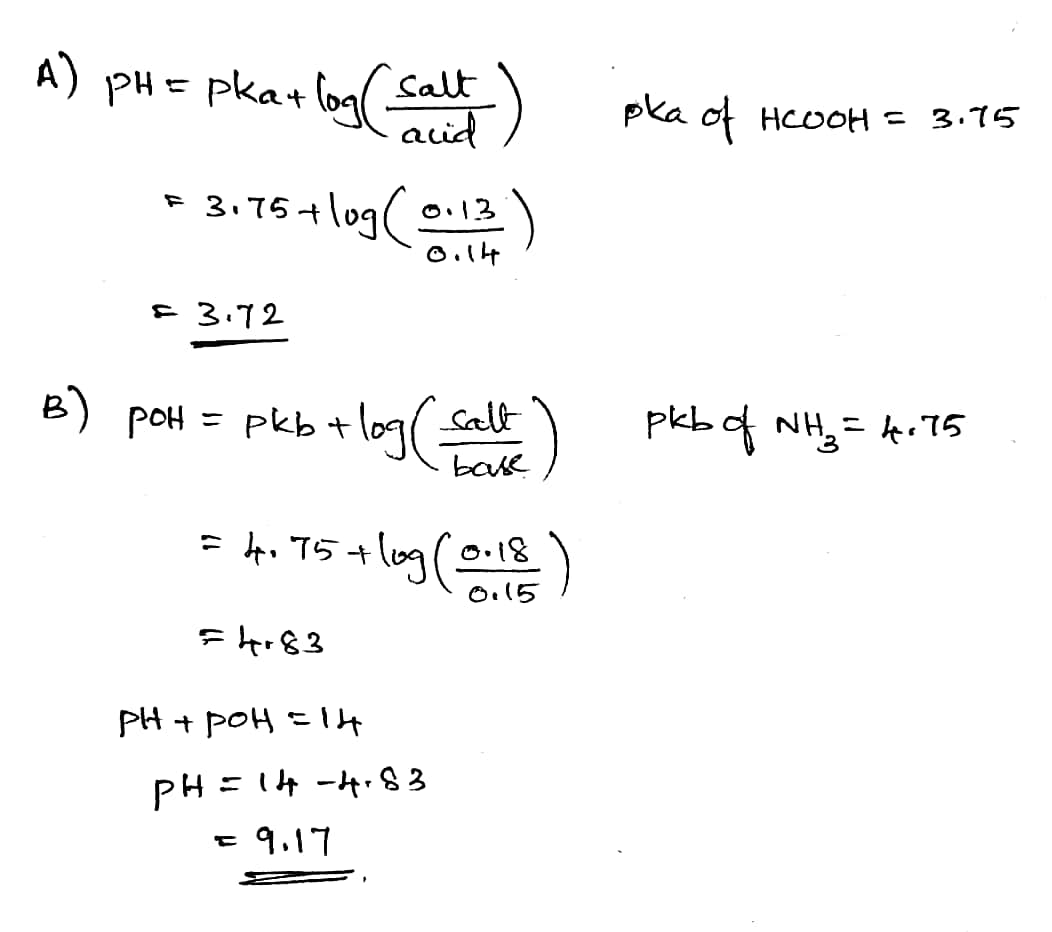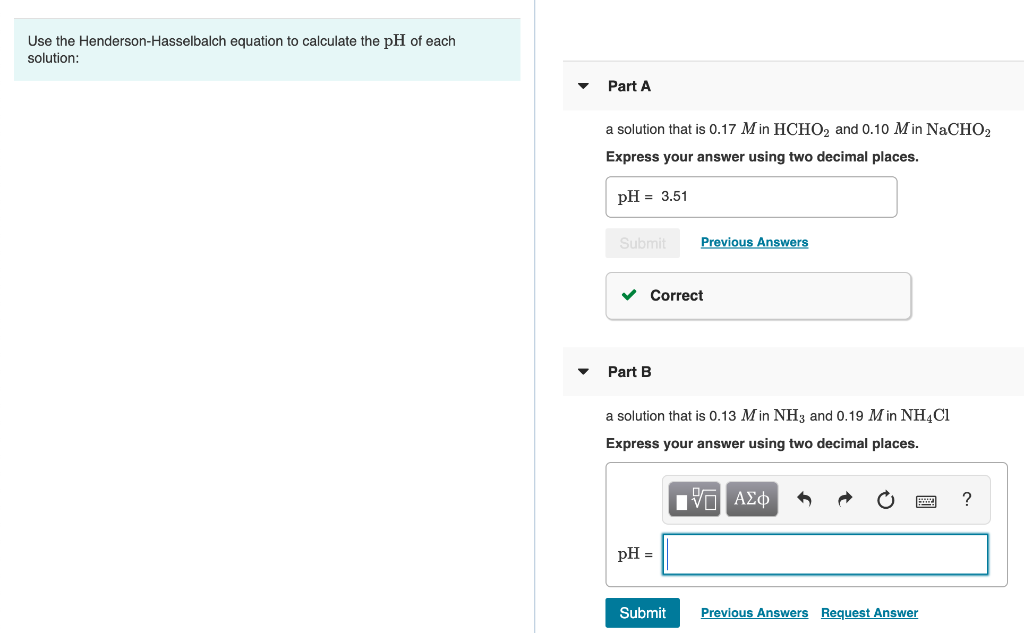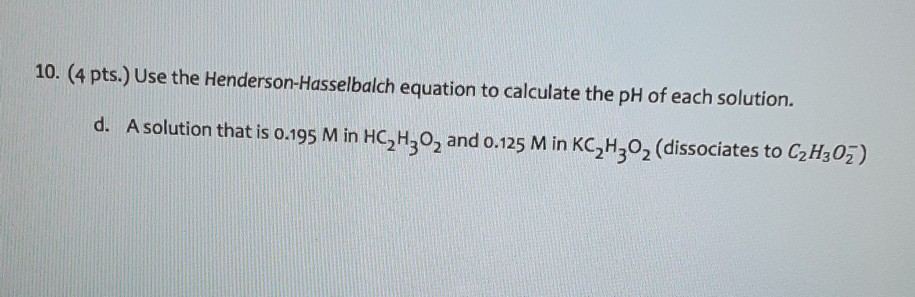# Use the Henderson-Hasselbalch equation to calculate the pH of each solution: A. a solution that is...

Use the Henderson-Hasselbalch equation to calculate the pH of each solution:

A. a solution that is 0.14 M in HCHO2 and 0.13 M in NaCHO2

B. a solution that is 0.15 M in NH3 and 0.18 M in NH4Cl#### Earn Coin

Coins can be redeemed for fabulous gifts.

Similar Homework Help Questions
• ### Use the Henderson-Hasselbalch equation to calculate the pH of each solution: A. a solution that is...

Use the Henderson-Hasselbalch equation to calculate the pH of each solution: A. a solution that is 0.14 M in HCHO2 and 0.13 M in NaCHO2 B. a solution that is 0.15 MM in NH3NH3 and 0.18 MM in NH4Cl

• ### Use the Henderson-Hasselbalch equation to calculate the pH of each solution: (a) a solution that is...

Use the Henderson-Hasselbalch equation to calculate the pH of each solution: (a) a solution that is 0.20 M in HCHO2 and 0.10 M in NaCHO2. (Ka=1.8×10−4) (b) a solution that is 0.14 M in NH3 and 0.19 M in NH4Cl. (Kb=1.76×10−5)

• ### Use the Henderson-Hasselbalch equation to calculate the pH of each solution: Part A a solution that...Use the Henderson-Hasselbalch equation to calculate the pH of each solution: Part A a solution that is 0.17 Min HCHO2 and 0.10 Min NaCHO2 Express your answer using two decimal places. pH = 3.51 Previous Answers ✓ Correct Part B a solution that is 0.13 Min NH3 and 0.19 M in NH4Cl Express your answer using two decimal places. I AM A O 2 ? pH = | Submit Previous Answers Request Answer

• ### Use the Henderson-Hasselbalch equation to calculate the pH of each solution: A solution that is 0.14...

Use the Henderson-Hasselbalch equation to calculate the pH of each solution: A solution that is 0.14 M in NH3 and 0.20 M in NH4Cl. (Kb= 1.76x10^-5)

• ### Solve an equilibrium problem (using an ICE table) to calculate the pH of of each solution:...

Solve an equilibrium problem (using an ICE table) to calculate the pH of of each solution: a solution that is 0.15 M in HCHO2 and 0.15 M in NaCHO2 and a solution that is 0.14 M in NH3 and 0.22 M in NH4Cl

• ### calculate pH using Henderson-Hasselbalch equation

Use the Henderson-Hasselbalch equation to calculate the pH of each of the following solutions.a. a solution that is 0.17 in and 0.11 in b. a solution that is 0.13 in and 0.19 in

• ### Use the Henderson-Hasselbalch equation to calculate the pHpH of each solution: Part A a solution that...

Use the Henderson-Hasselbalch equation to calculate the pHpH of each solution: Part A a solution that is 0.19 MM in HCHO2HCHO2 and 0.10 MM in NaCHO2

• ### 10. (4 pts. Use the Henderson-Hasselbalch equation to calculate the pH of each solution. d. A...10. (4 pts. Use the Henderson-Hasselbalch equation to calculate the pH of each solution. d. A solution that is 0.195 M in HC,H,, and 0.125 Min KC H202 (dissociates to C2H302)

• ### Using Henderson-Hasselbalch equation, Calculate the pH of a buffer solution that is 0.060 M formic acid...

Using Henderson-Hasselbalch equation, Calculate the pH of a buffer solution that is 0.060 M formic acid (HCHO2) and 0.150 M potassium formate (KCHO2). Remember that Ka = 1.8 X 10-4 for formic acid. Group of answer choices A 1.45 B 2.36 C.9.12 D.4.13 E. 0.0125 F. 7.00

• ### Use the Henderson-Hasselbalch equation to calculate the pH of each of the following solutions. A. a...

Use the Henderson-Hasselbalch equation to calculate the pH of each of the following solutions. A. a solution that contains 0.800% C5H5N by mass and 0.950% C5H5NHCl by mass (where pKa=5.23 for C5H5NHCl B. a solution that has 17.0 g g of HF and 27.0 g g of NaF in 125 mL m L of solution (where pKa=3.17 for HF acid)